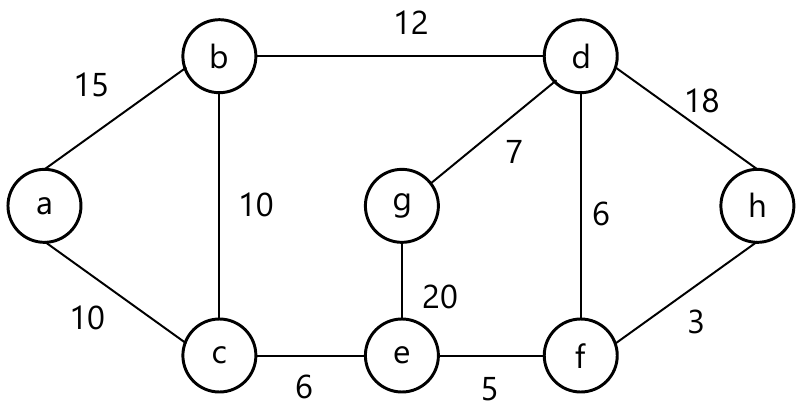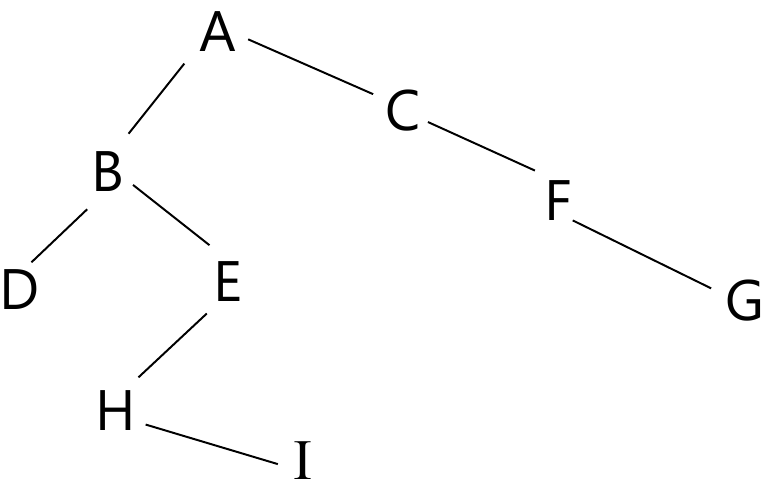MORE IN Data Structure & Algorithm Analysis
MU Information Technology (Semester 3)
Data Structure & Algorithm Analysis
May 2014
Total marks: --
Total time: --
INSTRUCTIONS
(1) Assume appropriate data and state your reasons
(2) Marks are given to the right of every question
(3) Draw neat diagrams wherever necessary

1 (a) What is stack? Give application of it.
2 M
1 (b) What is time complexity? Determine time complexity of following code:-
for (i=1; i<=n; i++)
for (j=1; j<=n; j++)
x=x+1;
3 M
1 (c) Explain with e.g. :-
(i) Complete binary tree
(ii) Degree of tree
(iii) Height of tree
3 M
1 (d) Explain linked list with its various types.
3 M
1 (e) Define double ended queue and give its applications.
3 M
1 (f) Define asympotic notation along with example.
3 M
1 (g) Define Graph List its types with example.
3 M

2 (a) Find the shorted path using Dijkstra's algorithm10 M
2 (b) Implement quick sort with example and find its complexity
10 M

3 (a) Explain BFS and DFS with algorithm and proper example.
10 M
3 (b) What is linked list? Write 'C' function for insertion of 'n' elements.
10 M

4 (a) Traverse the following binary tree into preorder, inorder, postorder by giving its algorithm10 M
4 (b) Using Prim's algorithm find minimum spanning tree of a graph with example. Write algorithm of it.
10 M

5 (a) What is priority queue? Give implementation of it.
10 M
5 (b) What is graph? Give representation of graph with example. State applications of it.
10 M

Solve any four :-
6 (a) AVL Tree
5 M
6 (b) Euclids algorithm
5 M
6 (c) Sparse matrix
5 M
6 (d) B-Tree
5 M